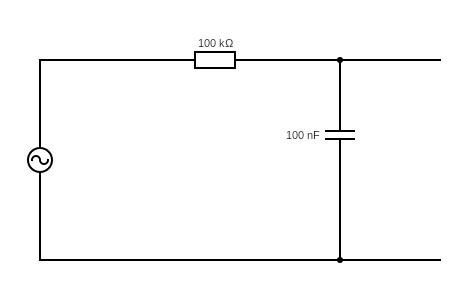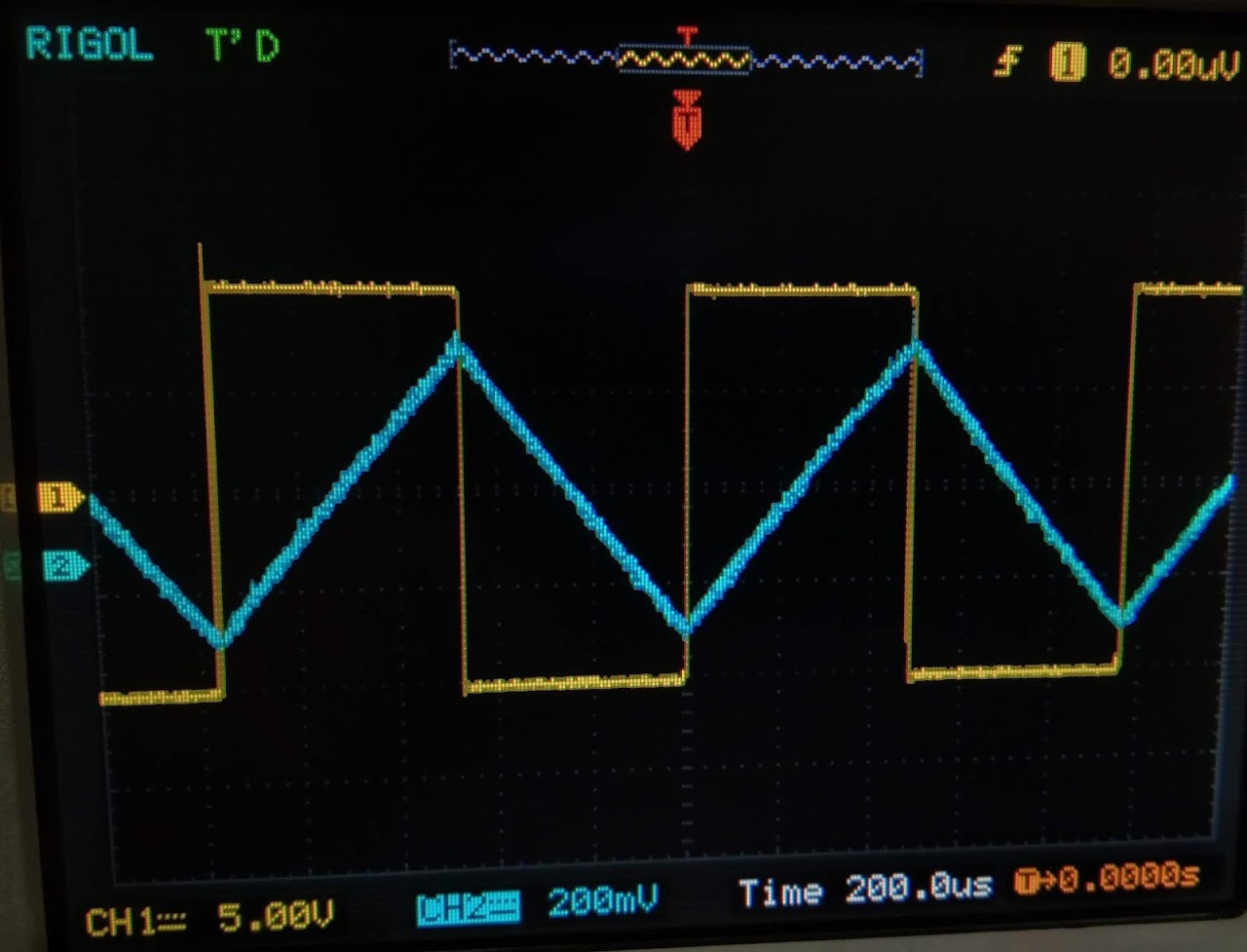Integrator circuit

Integrator circuit

Aim:-

To construct and study Integrator circuit

Apparatus:

$0.005\mu F$ Capacitor,$4.7 K\ohm$ Resistor, Digital Storage Oscilloscope, Function Generator, 2 mm Patch cords, Breadboard,Connecting Wires

Take a breadboard and Connect the circuit see in figure  Take a function generator and Give AC signal 10 V,500 Hz Sinusoidal waveform Conect the input and output signal of circuit in Digital Storage Oscilloscope Observe input signal and output signal (adsbygoogle = window.adsbygoogle || []).push({});(1) Sinusodal wave(2) Triangular Wave(3) Rectangular WaveLaTeX4Web 1.4 OUTPUTPhase angle between input signal and output signal
tanq =(1)/(2p fRC)
tanq max=¥

q=90
RC =102

if frequency increase then q decrease
if frequency decrease then q increase

Result

So,we can easily say from observations that integration circuit gives output waveform which is integration of input waveform, frequency decrease integration becomes poorer and poorer